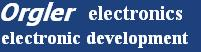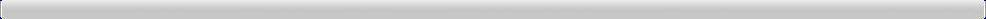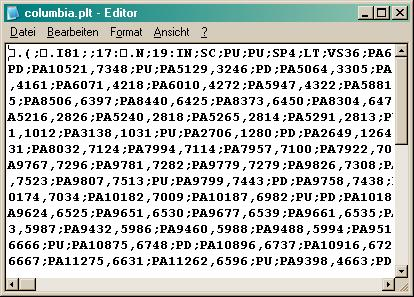Plot Columbia# 1. Plot HPGL-Data into a MFC SDI window# 2. Plot commands

PU; Pen up
PD; Pen down
PA5064,3305; Plot absolute 5064 mils x_axis and 3305 mils y_axis

# 3. Open data file

```void CHpglDoc::Serialize(CArchive& ar)
{
if (ar.IsStoring())
{
// TODO: add storing code here
}
else
{
}
}
```

# (

```//Set the pointers
ptr1       = &cBuffer;
ptr1end   = ptr1 + nFileSize;      //Pointers are set

while(ptr1 < ptr1end)
{
ptr2 = &cLine;   			// ptr2 on top of the small memory

while(*ptr1 != ';') *ptr2++ = *ptr1++;	//  while ptr1 unequal ';'

ptr1++;   				// jump over the semicolon
*ptr2 = NULL;  //end of string

// ====data are in the small memory====
if(cLine=='P')           	// evaluation only when the first letter is a 'P'
{
if(cLine== 'U') penx = 0; 	//if the first letter is an 'U' pen x = 0 (move to)
if(cLine== 'D') penx = 1;	//if the first letter is an 'D' pen x = 1 (line to)
if(cLine== 'A')		//if the first letter is an 'A' the linescan can begin
{
sscanf(cLine,"PA%d,%d", &aa, &bb);  aa/=40;  bb/=40;//scan the line(line,"how exactly looks the line",make aa & bb looks like the coordinats)
xx=350-aa;			//move the picture in x-direction
yy=240-bb;			//move the picture in y-direction
if(penx == 0) pDC->MoveTo(xx,yy);   //move to the coordinats xx,yy only when penx=0
else pDC->LineTo(xx,yy);     //line to the coordinats xx,yy only when penx=1

}//end when 'A'
}//end when first letter = 'P'
}// End of ptr1
```